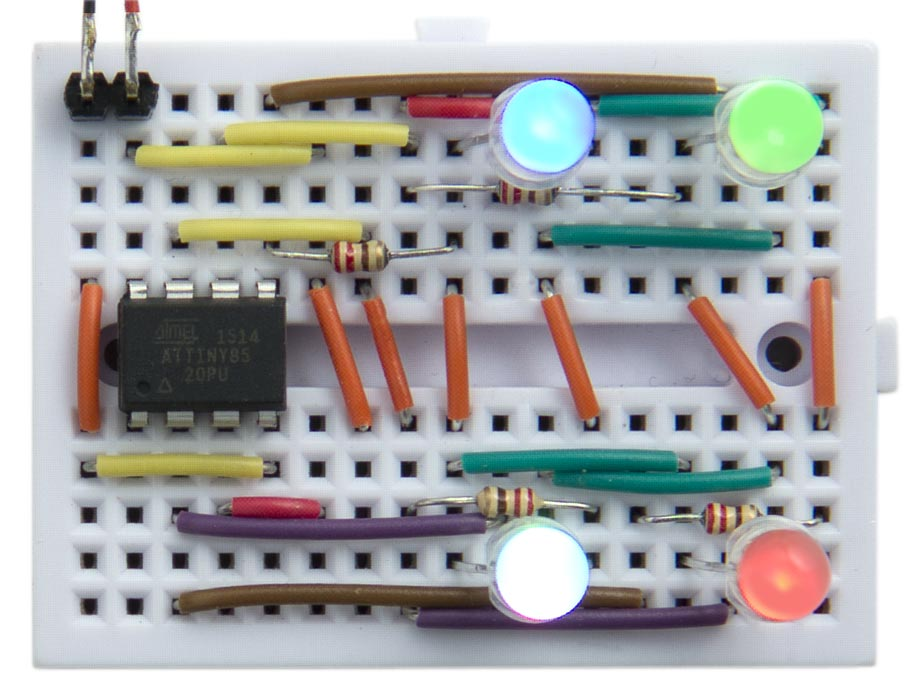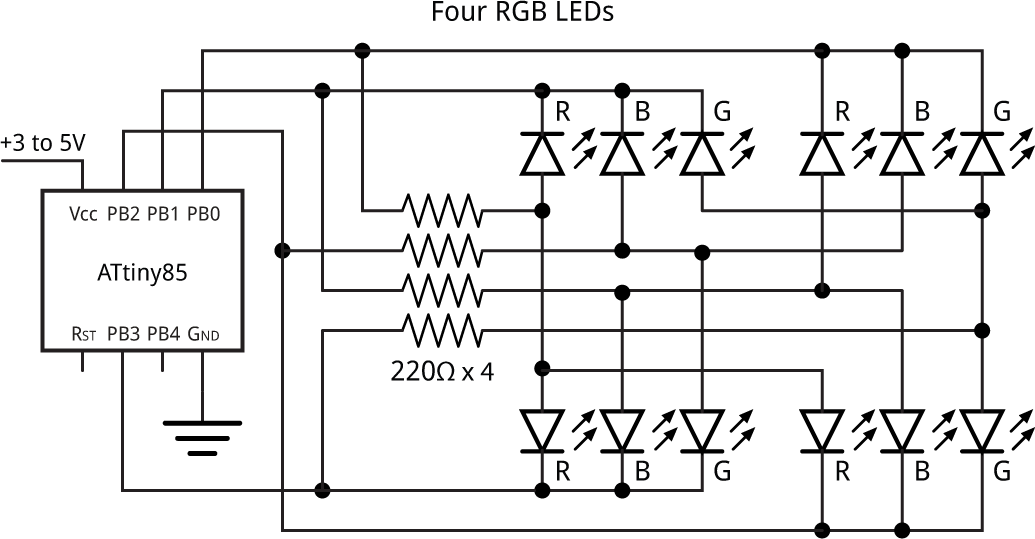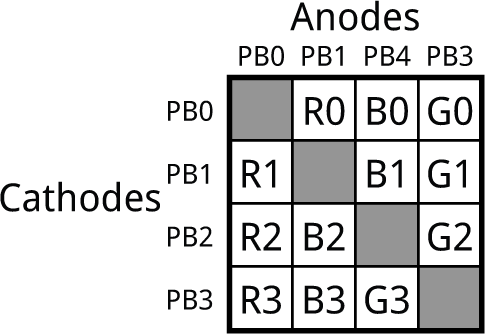## Driving Four RGB LEDs from an ATtiny85

17th May 2017

This is a simple application showing how to drive four RGB LED packages from an ATtiny85:Driving four RGB LED packages from an ATtiny85.

Each LED can be set to one of 16 different levels, from off to full brightness, and the circuit leaves one I/O pin free for another application.

### The circuit

Here's the circuit:Circuit for driving four RGB LED packages from an ATtiny85.

The application takes advantage of the fact that you can drive 12 LEDs from four I/O lines using charlieplexing. The following table shows which LED lights up in each RGB package when you take one I/O line high and the other I/O line low:It's compatible with common-cathode RGB LED packages; I used low-cost four-lead common-cathode 5mm RGB LEDs , but you should be able to use any sort of RGB LEDs.

### Display multiplexing

The LEDs are updated in the background, from the values in Buffer[], using interrupts generated by Timer/Counter1, leaving Timer/Counter0 free for use by delay() and millis().

Each element of the array Buffer[] determines the colour and brightness of one RGB LED. The value is specified as three hexadecimal digits, for the colours BGR. A value of 0 specifies zero brightness, and a value of 'F' specifies full brightness. For example:

`Buffer = 0xF00; Buffer = 0x0F0; Buffer = 0x00F; Buffer = 0x888;`

sets the four LEDs to 100% green, 100% blue, 100% red, and 50% white respectively.

The timer is set up in the routine DisplaySetup() for CTC mode, counting up to OCR1C:

```void DisplaySetup () {
// Set up Timer/Counter1 to multiplex the display
TCCR1 = 1<<CTC1 | 7<<CS10;    // CTC mode; divide by 64
OCR1C = 24;                   // Divide by 25 -> 5kHz
TIMSK = TIMSK | 1<<OCIE1A;    // Enable overflow interrupt
}```

The Timer/Counter1 Compare A interrupt displays the next row of LEDs:

```ISR(TIM1_COMPA_vect) {
DisplayNextRow();
}```

For each RGB LED package the routine DisplayNextRow() is called 16 times. The brightness value of the R, G, and B components for the specified LED package are read from the value in Buffer[led], and these then determine how many of the 16 cycles the colour will be lit for:

```void DisplayNextRow() {
static int cycle = 0;
DDRB = DDRB & ~(1<<(cycle & 0x03));
cycle = (cycle + 1) & 0x3F;   // 64 cycles
int led = cycle & 0x03;
int count = cycle>>2;
int rgb = Buffer[led];
int r = rgb & 0x0F;
int b = rgb>>4 & 0x0F;
int g = rgb>>8 & 0x0F;
int bits = (count < r) | (count < b)<<1 | (count < g)<<2;
bits = bits + (bits & 0x07<<led);
DDRB = (DDRB & 0xF0) | bits;
PORTB = (PORTB & 0xF0) | bits;
DDRB = DDRB | 1<<led;
}```

The interrupt occurs at a rate of 5kHz, so a complete set of 64 cycles of DisplayNextRow() occurs at approximately 78Hz, fast enough to avoid flicker.

### Demonstration program

The following demonstration program in loop() slowly cycles the LEDs through all possible colours and brightnesses:

```int Step = 0;

int red (int x) {
int y = x % 48;
if (y > 15) y = 31 - y;
return max(y, 0);
}

int green (int x) { return red(x + 32); }
int blue (int x) { return red(x + 64); }

void loop () {
for (int i=0; i<4; i++) {
Buffer[i] = green(Step + i*12)<<8 | blue(Step + i*12)<<4 | red(Step + i*12);
}
Step++;
delay(200);
}```

### Compiling the program

Compile the program using the ATTiny Core, which supports all the ATtiny processors and supersedes the various earlier ATtiny cores . Choose the ATtiny25/45/85 option under the ATtinyCore heading on the Board menu. Then choose Timer 1 Clock: CPUB.O.D. Disabled, ATtiny85, 8 MHz (internal) from the subsequent menus. Choose Burn Bootloader to set the fuses appropriately. Then upload the program to the ATtiny85.

Here's the whole Four RGB LED program: Four RGB LED Program.

1. ^ Kingbright RGB Through Hole 5mm LEDs on Farnell.
2. ^ ATTinyCore on GitHub.